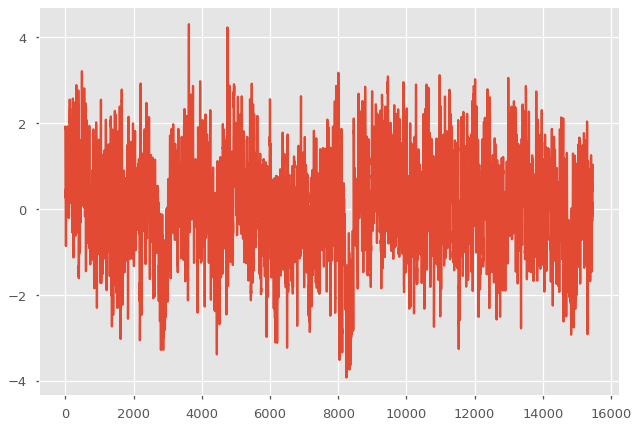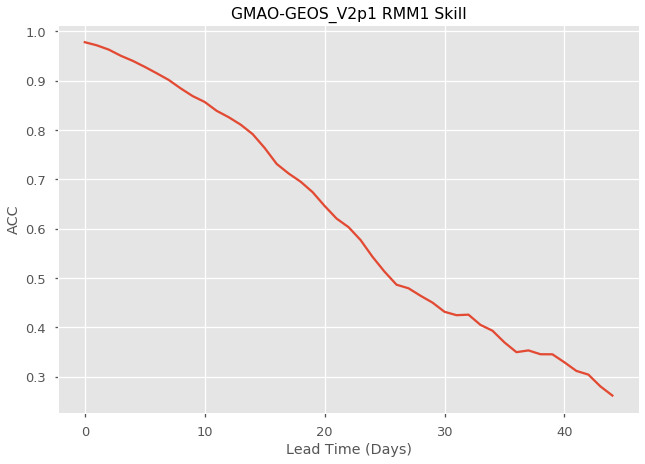# Calculate the skill of a MJO Index as a function of lead time¶

## In this example, we demonstrate:¶

1. How to remotely access data from the Subseasonal Experiment (SubX) hindcast database and set it up to be used in climpred.

2. How to calculate the Anomaly Correlation Coefficient (ACC) using daily data with climpred

3. How to calculate and plot historical forecast skill of the real-time multivariate MJO (RMM) indices as function of lead time.

## The Subseasonal Experiment (SubX)¶

Further information on SubX is available from Pegion et al. 2019 and the SubX project website

The SubX public database is hosted on the International Research Institute for Climate and Society (IRI) data server http://iridl.ldeo.columbia.edu/SOURCES/.Models/.SubX/

Since the SubX data server is accessed via this notebook, the time for the notebook to run may is several minutes and will vary depending on the speed that data can be downloaded. This is a large dataset, so please be patient. If you prefer to download SubX data locally, scripts are available from https://github.com/kpegion/SubX.

## Definitions¶

RMM

Two indices (RMM1 and RMM2) are used to represent the MJO. Together they define the MJO based on 8 phases and can represent both the phase and amplitude of the MJO (Wheeler and Hendon 2004). This example uses the observed RMM1 provided by Matthew Wheeler at the Center for Australian Weather and Climate Research. It is the version of the indices in which interannual variability has not been removed.

Skill of RMM

Traditionally, the skill of the RMM is calculated as a bivariate correlation encompassing the skill of the two indices together (Rashid et al. 2010; Gottschalck et al. 2010). Currently, climpred does not have the functionality to calculate the bivariate correlation, thus the anomaly correlation coefficient for RMM1 index is calculated here as a demonstration. The bivariate correlation metric will be added in a future version of climpred

:

import warnings

import matplotlib.pyplot as plt
plt.style.use('ggplot')
plt.style.use('seaborn-talk')

import xarray as xr
import pandas as pd
import numpy as np

from climpred import HindcastEnsemble
import climpred

:

warnings.filterwarnings("ignore")


Function to set 360 calendar to 360_day calendar and decond cf times

:

def decode_cf(ds, time_var):
if ds[time_var].attrs['calendar'] == '360':
ds[time_var].attrs['calendar'] = '360_day'
ds = xr.decode_cf(ds, decode_times=True)
return ds


:

obsds = climpred.tutorial.load_dataset('RMM-INTERANN-OBS')['rmm1'].to_dataset()
obsds = obsds.dropna('time') # Get rid of missing times.

:

plt.plot(obsds['rmm1'])

:

[<matplotlib.lines.Line2D at 0x7fc0f922b9b0>]Read the SubX RMM1 data for the GMAO-GEOS_V2p1 model from the SubX data server. It is important to note that the SubX data contains weekly initialized forecasts where the init day varies by model. SubX data may have all NaNs for initial dates in which a model does not make a forecast, thus we apply dropna over the S=init dimension when how=all data for a given S=init is missing. This can be slow, but allows the rest of the calculations to go more quickly.

Note that we ran the dropna operation offline and then uploaded the post-processed SubX dataset to the climpred-data repo for the purposes of this demo. This is how you can do this manually:

url = 'http://iridl.ldeo.columbia.edu/SOURCES/.Models/.SubX/.GMAO/.GEOS_V2p1/.hindcast/.RMM/.RMM1/dods/'
fcstds = xr.open_dataset(url, decode_times=False, chunks={'S': 1, 'L': 45}).dropna(dim='S',how='all')

:

fcstds = climpred.tutorial.load_dataset('GMAO-GEOS-RMM1', decode_times=False)
print(fcstds)

<xarray.Dataset>
Dimensions:  (L: 45, M: 4, S: 510)
Coordinates:
* S        (S) float32 14245.0 14250.0 14255.0 ... 20439.0 20444.0 20449.0
* M        (M) float32 1.0 2.0 3.0 4.0
* L        (L) float32 0.5 1.5 2.5 3.5 4.5 5.5 ... 40.5 41.5 42.5 43.5 44.5
Data variables:
RMM1     (S, M, L) float32 ...
Attributes:
Conventions:  IRIDL


The SubX data dimensions correspond to the following climpred dimension definitions: X=lon,L=lead,Y=lat,M=member, S=init. We will rename the dimensions to their climpred names.

:

fcstds=fcstds.rename({'S': 'init','L': 'lead','M': 'member', 'RMM1' : 'rmm1'})


Let’s make sure that the lead dimension is set properly for climpred. SubX data stores leads as 0.5, 1.5, 2.5, etc, which correspond to 0, 1, 2, … days since initialization. We will change the lead to be integers starting with zero.

:

fcstds['lead']=(fcstds['lead']-0.5).astype('int')


Now we need to make sure that the init dimension is set properly for climpred. For daily data, the init dimension must be a xr.CFTimeIndex or a pd.DatetimeIndex. We convert the init values to pd.DatetimeIndex.

:

fcstds = decode_cf(fcstds, 'init')


climpred also requires that lead dimension has an attribute called units indicating what time units the lead is assocated with. Options are: years,seasons,months,weeks,pentads,days. For the SubX data, the lead units are days.

:

fcstds['lead'].attrs={'units': 'days'}


Create the climpred HindcastEnsemble object and add the observations.

:

hindcast = HindcastEnsemble(fcstds)

:

hindcast = hindcast.add_observations(obsds, 'obs')


Calculate the Anomaly Correlation Coefficient (ACC)

:

skill = hindcast.verify(metric='acc')


Plot the skill as a function of lead time

:

plt.plot(skill['rmm1'])
plt.title('GMAO-GEOS_V2p1 RMM1 Skill')

:

Text(0, 0.5, 'ACC')